# Physics Assignment Help With Power

## Power Assignment HelpPower is defined as the rate at which work is done. If an amount of work DW is done in a time interval Dt, then the average power is defined to be
Pav =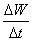The SI unit of power is J/s which is given the name watt (W) in the honour of James Watt.

Thus, 1 W = 1 J/s.

The instantaneous power is the limiting value of Pav as Dt ® 0; that is
P =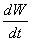The work done by force F on a object that has an infinitesimal displacement d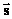is

dW =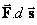Since the velocity of the object is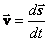, the instantaneous power may be written as P ==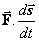or P =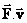Since the work and energy are closely related, a more general definition of power is the rate of energy transfer from one body to another, or the rate at which energy is transformed from one form to another.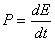### Assignment Help For Power

assignmenthelp.net provides best Online Assignment Help service in Physics for all standards. Our Tutor provide their high quality and optimized Tutorial help to fulfill all kind of need of Students.